# A Second Look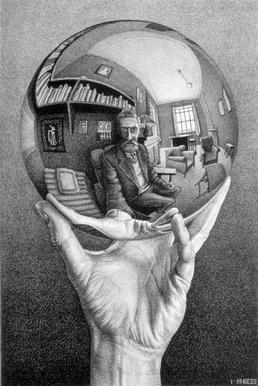M.C. Escher’s 1935 lithograph Hand With Reflecting Sphere gave artist Kelly M. Houle an idea.

She drew this image in charcoal on a piece of illustration board: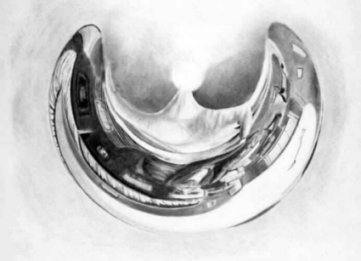Now when a cylindrical mirror is placed at the center, it produces this reflection: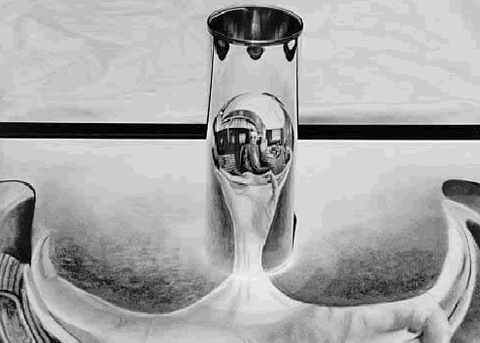“When the original image is bent and stretched into a circular swath, the shadows seem to fall in all directions,” she wrote. “When the curved mirror is used to reflect the anamorphic distortion, the forms take on the familiar rules of light and shading that make them seem three-dimensional.”

(Kelly M. Houle, “Portrait of Escher: Behind the Mirror,” in D. Schattschneider and M. Emmer, eds., M.C. Escher’s Legacy, 2003.)

# Escalating Powers$\displaystyle 1 + 5 + 10 + 24 + 28 + 42 + 47 + 51 = 2 + 3 + 12 + 21 + 31 + 40 + 49 + 50\newline 1^{2} + 5^{2} + 10^{2} + 24^{2} + 28^{2} + 42^{2} + 47^{2} + 51^{2} = 2^{2} + 3^{2} + 12^{2} + 21^{2} + 31^{2} + 40^{2} + 49^{2} + 50^{2}\newline 1^{3} + 5^{3} + 10^{3} + 24^{3} + 28^{3} + 42^{3} + 47^{3} + 51^{3} = 2^{3} + 3^{3} + 12^{3} + 21^{3} + 31^{3} + 40^{3} + 49^{3} + 50^{3}\newline 1^{4} + 5^{4} + 10^{4} + 24^{4} + 28^{4} + 42^{4} + 47^{4} + 51^{4} = 2^{4} + 3^{4} + 12^{4} + 21^{4} + 31^{4} + 40^{4} + 49^{4} + 50^{4}\newline 1^{5} + 5^{5} + 10^{5} + 24^{5} + 28^{5} + 42^{5} + 47^{5} + 51^{5} = 2^{5} + 3^{5} + 12^{5} + 21^{5} + 31^{5} + 40^{5} + 49^{5} + 50^{5}\newline 1^{6} + 5^{6} + 10^{6} + 24^{6} + 28^{6} + 42^{6} + 47^{6} + 51^{6} = 2^{6} + 3^{6} + 12^{6} + 21^{6} + 31^{6} + 40^{6} + 49^{6} + 50^{6}\newline 1^{7} + 5^{7} + 10^{7} + 24^{7} + 28^{7} + 42^{7} + 47^{7} + 51^{7} = 2^{7} + 3^{7} + 12^{7} + 21^{7} + 31^{7} + 40^{7} + 49^{7} + 50^{7}$

# For the Record

Western Kentucky University geoscientist John All was traversing Nepal’s Mount Himlung in May 2014 when the ice collapsed beneath him and he fell into a crevasse, dislocating his shoulder and breaking some ribs. He landed on a ledge, but now he faced a 70-foot climb back to the surface alone without the use of his right arm or upper leg.

“That’s when I pulled my research camera out and started talking to myself about all my options,” he told National Geographic. “I take photos of everything I do because, if I’m working in Africa and I need to recall a detail, that’s going to be the best way to do it. I was also thinking about my mom and my friends and family and realized that just talking wouldn’t convey what was happening to me nearly as well. So I started recording things.”

“It probably took me four or five hours to climb out,” he said. “I kept moving sideways, slightly up, sideways, slightly up, until I found an area where there was enough hard snow that I could get an ax in and pull myself up and over. I knew that if I fell at any time in that entire four or five hours, I, of course, was going to fall all the way to the bottom of the crevasse. Any mistake, or any sort of rest or anything, I was going to die.”

After reaching the top he rolled as much as walked back to his tent, called for help, and waited 16 hours for a helicopter to arrive. He wrote later, “I had dug myself out of my own grave.”

# Math Notes

121213882349 = (1212 + 1388 + 2349)3

128711132649 = (1287 + 1113 + 2649)3

162324571375 = (1623 + 2457 + 1375)3

171323771464 = (1713 + 2377 + 1464)3

368910352448 = (3689 + 1035 + 2448)3

# Alchemy

Mike Keith found this amazing correspondence in 2004. The two 6×6 squares below contain 72 different entries from the periodic table of the elements: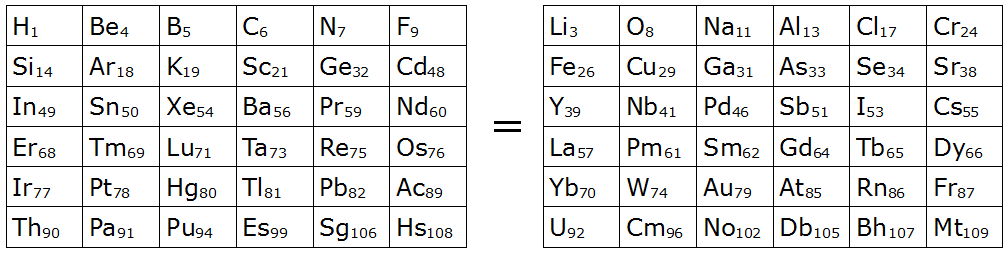The two squares are equal in three different ways:

1. If you spell out the name of each element listed (hydrogen, beryllium, etc.), the square on the left is an anagram of the square on the right.
2. The sum of the atomic numbers of the 36 elements on the left (2019) equals the sum of those on the right.
3. If you replace each symbol with its alphabetic score (where A=1, B=2, etc.; e.g. Li = L + I = 12 + 9 = 21), then the sum of the scores on the left (737) equals that of those on the right.

Keith writes, “The next largest pair of triply-equal squares like this would be 7×7 in size, containing a total of 98 different elements, [and] it seems quite unlikely that 98 of them could be so arranged. If this is true then the 6×6 pair presented here is the largest possible (at least for now, until many more new chemical elements have been discovered and named).”

(Mike Keith, “A Magical Pair of 6×6 Chemical Squares,” Word Ways, February 2004.)

# Sylver Coinage

This curious game was invented by Princeton mathematician John H. Conway. Two players take turns naming positive integers, but an integer is off limits if it’s the sum of nonnegative multiples of integers that have already been named. Once 1 is named, everything is off limits (because any positive integer is a sum of 1s), so that ends the game; the player who is forced to name 1 is the loser. An example gives the idea:

• I start by naming 5. From now on neither of us can name 5, 10, 15, 20, …
• You name 4. Now neither of us can name a number built of 5s and 4s, that is, 4, 5, 8, 9, 10, or any number greater than 11.
• I name 11. This reduces the list of available numbers to 1, 2, 3, 6, and 7.
• You name 6. Now we’re down to 1, 2, 3, and 7.
• I name 7. Only 1, 2, and 3 are still available to name.

Now we’ll use our next turns to name 2 and 3, and you’ll be forced to name 1, losing the game.

Though the game is very easy to understand, it’s still full of mysteries. For example, R.L. Hutchings has shown that playing a prime number as the first move guarantees that a winning strategy exists, but no one has figured out how to find the strategy. And no one knows whether there are any winning opening moves at all that aren’t prime.

More on the Sylver Coinage page.

Here’s an especially vivid example of the illusion created by Dick Termes’ six-point perspective.

If you can convince yourself that the front half of this sphere is transparent, and that the image is painted on the interior of the back half, you’ll find that you’re inside the cage, turning to your left, while the birds are outside the cage, looking in at you. (To get started, I find it helps to focus on an edge of the sphere, rather than the center.)

There are many more examples on Termes’ YouTube channel.

# The Last Detail

A puzzle by R.P. Cross:

Find the last digit in the evaluation of$\sum_{n = 1}^{100}n!$

# Podcast Episode 164: Vigil on the Ice

In 1930, British explorer Augustine Courtauld volunteered to spend the winter alone on the Greenland ice cap, manning a remote weather station. As the snow gradually buried his hut and his supplies steadily dwindled, his relief party failed to arrive. In this week’s episode of the Futility Closet podcast we’ll follow Courtauld’s increasingly desperate vigil on the ice.

We’ll also retreat toward George III and puzzle over some unexpected evidence.

See full show notes …

# A Good Start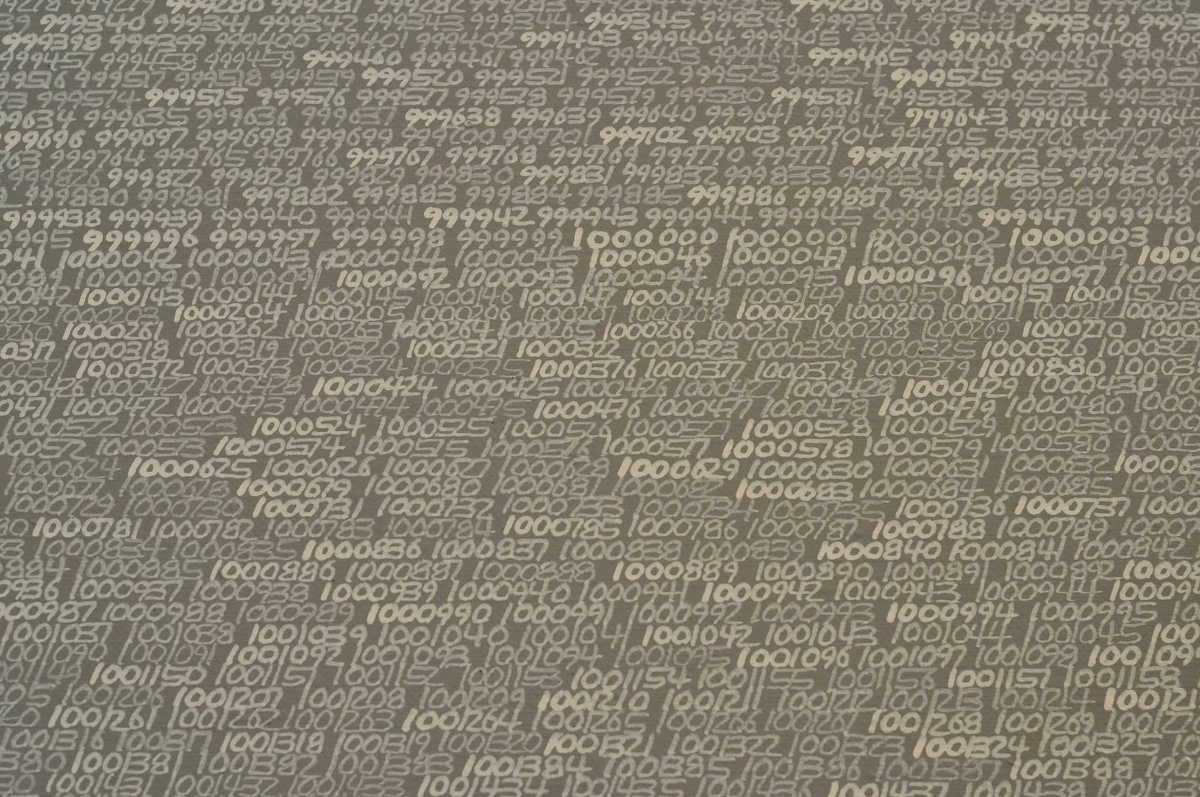In 1965, Polish artist Roman Opałka hung a 196 × 135 cm canvas in his Warsaw studio. In the top left corner he painted a tiny numeral 1, then a 2, and so on until he had filled the canvas with numbers. Then he put up a new canvas and continued where he had left off. He called these images “details”; all of them had the same size and the same title, 1965 / 1 – ∞.

He vowed to spend the rest of his life on the project. “All my work is a single thing,” he said, “the description from number one to infinity. A single thing, a single life. … The problem is that we are, and are about not to be.”

At the start he painted white numbers on a black background, but in 1972 he began gradually to lighten the black with each detail, saying that his goal was “to get up to the white on white and still be alive.” He expected that this would happen when he reached 7777777 … but at the time of his death, in 2011, he’d got only as far as 5607249.